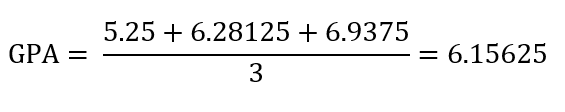# How Does GEMSAS Interpret Your GPA?

by , 31 March, 2022

As you are likely aware, your GPA is an important component of your Australian graduate medical school application. However, you may not be aware of the details of the rather confusing process that GEMSAS uses to calculate your GPA. It may be different to how your university calculates your GPA! The good news is, GEMAS provides information on how does GEMSAS interpret your GPA on their website. The bad news is that this information can feel quite wordy and overwhelming to take in at first. Today we’ll go through the GEMSAS GPA calculation guide step-by-step, and hopefully demystify things!

## What exactly is a GPA?A GPA, or Grade Point Average, is a numerical way of converting your grades (HD, D, C, P) into an average score. GEMSAS, and many Australian universities, use a 7-point scale. Under this scale, HD is usually a 7, D a 6, C a 5, P a 4, and failing a 0. The GPA is then the average of these scores.

However, since different universities use different cut-offs for their grades (e.g. at UWA, 80% is an HD, whereas at UNSW, you need 85% to get an HD), GEMSAS prefers to convert from your percentage score wherever possible in order to standardise things across the different universities. This means that a score of 80% or above will give you a subject GPA of 7—good news for those of you getting 83% at UNSW!

Some universities use an unweighted GPA, in which case your overall GPA is simply the average of your subject GPAs. However, other universities use a weighted GPA, where final year results get weighted by a factor of 3, second last year by a factor of 2, and the third last year by a factor of 1 (i.e. your final year results are more important than your earlier results). Check the GEMSAS Admissions Guide to find out which formula is used by the medical school you are applying to.

## Which subjects are valid?

When calculating your GPA, you first need to ask yourself which subjects will be used in the calculation. You have probably heard that the last 3 years of full-time study are used in the calculation. If you’ve only done a full-time Bachelor’s degree, this is really easy to figure out: the last three years of your degree will count (so if you did a three-year degree, your whole degree will count, and if you did a four-year degree, your first year won’t count but all subsequent years will).

However, things can get more complicated if you have done other degrees. If you have done multiple Bachelor’s degrees, then your most recent degree will be considered. If you have done postgraduate qualifications, you will need to check the GEMSAS Admissions Guide, as not all universities will count postgraduate qualifications towards the GPA calculation. On the other hand, some universities provide bonuses for applicants who have completed a postgraduate degree. If you’ve somehow managed to do some exchange study in the past few years, you will need to look in the GEMSAS Admissions Guide to find out whether those results will be included.

Calculation can also be complicated if you did not study full-time. For the purposes of the calculation, “one year” is not a calendar year, but rather a year’s worth of credit points. So, if the university that you went to gives 12.5 credit points per unit, with a full-time study load of 8 units (100 credit points), your “final year” will be your last 100 credit points’ worth of units. For example, if you did 6 units per year over 4 years, your “final year” will be the 6 units (75 credit points) in your fourth year, plus 2 units (25 credit points) from the second semester of your third year. Which two units, you may ask? The system will advantage you by allocating your best two units from that semester to your “final year” for calculation purposes.

Due to the disruptions from COVID-19, some universities are not including 2020 results in the calculation. Others, however, may include any results where you were given a grade. Your best bet is to check the GEMSAS Admission Guide for the details for the medical school that you’re applying to.

Note that subjects you have failed will unfortunately be included in the calculation, and if you repeat a subject, then the result from both attempts will be included, provided both attempts fall within the final three years of study. (This, however, does not apply if you passed the same unit multiple times—in that case, only your first attempt will be included.)

GEMSAS includes several tools for you to calculate your GPA. The first of these tools is the GPA calculator. The other tool is this guide on how does GEMSAS interpret your GPA. Let’s go through this information together:

STEP ONE: Group subjects into GPA years. This occurs based on what was discussed above – if you have a three year Bachelor’s degree without any failed subjects, it should be easy. If your course was over a total of 3 years (full time), then just use the last 3 years for the calculation. If you were studying part time over multiple years, group your subjects by credit points to figure out which subjects constitute each “year” of study.

STEP TWO: Enter points. Now that you’ve got a chronological list of subjects completed, put your result next to each course, as well as credit points/unit values, and subject code. Preferably use percentages, and convert the grade to the GPA in Appendix A here.

STEP THREE: Calculate each year’s GPA. Multiply each unit’s GPA by the number of credit points that that unit is worth. Do this for all subjects in one year and add the results together to get a total for the year. Divide this total by the number of credit points in one year of full-time study at your institution. This should give you your overall GPA for that year! Repeat the process for all three years of study that will be included in the overall calculation.

STEP FOUR: Calculate overall GPA. This is different depending on whether you are applying somewhere with a weighted or unweighted GPA. For a weighted GPA, you will need to use the following formula: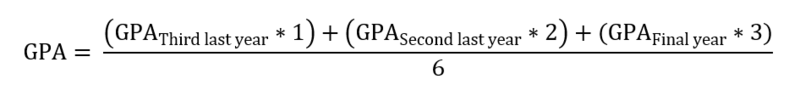For an unweighted GPA, the formula is as follows: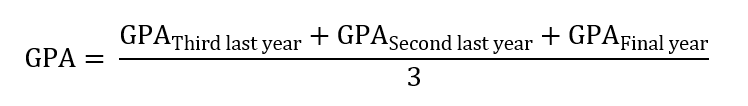## Example

This is an example of how to calculate your GPA for a part-time course over 5 years, which is a tricky situation. Hopefully, this will demonstrate to a wide variety of applicants what calculations go into getting to the GEMSAS GPA. In this example, we have a student going to a hypothetical university that has a full-time study load of 80 credit points per year (10 credit points per semester). The below table shows subject name, credit points, university grade, and converted grade (according to the table in Appendix A of GEMSAS’s guide. Note that in this hypothetical example, the university is in Column B).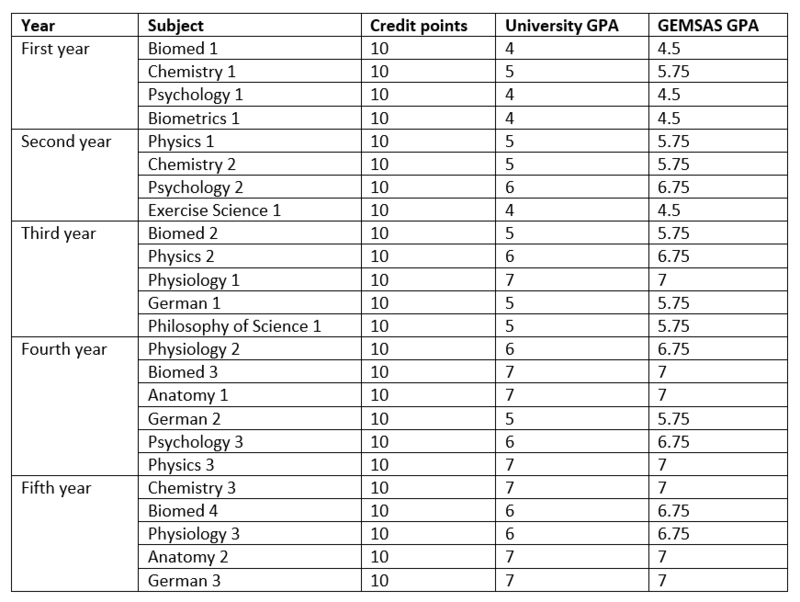The first step is to find the last three “full time” years. This can be done by grouping the subjects by “credit point” years, starting from the most recent study and working backwards.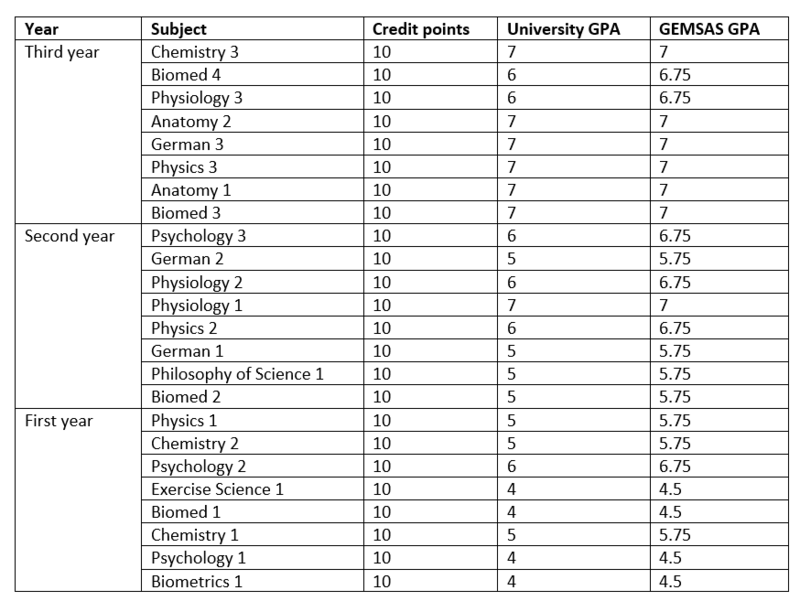The next step is to multiply each subject’s GPA by credit points, and then sum these up to get a year total: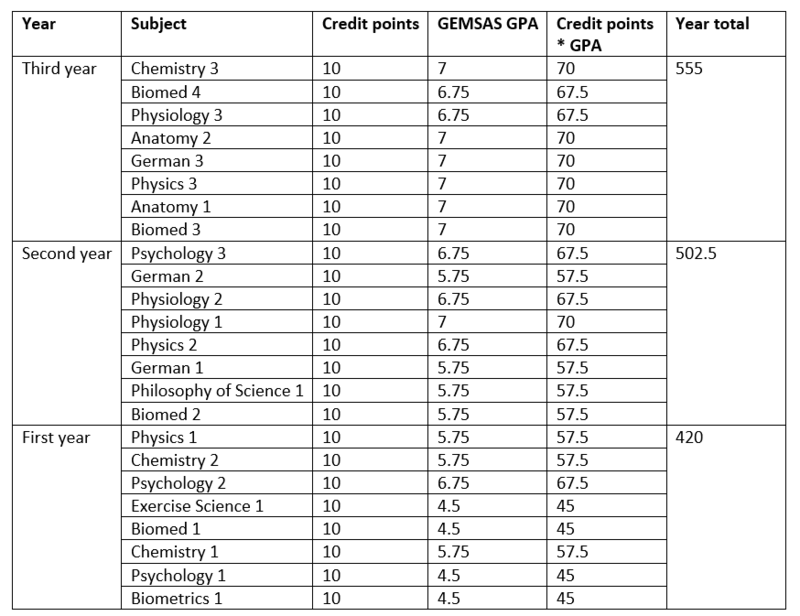The next step is to divide each year total by the number of credit points in a year, which is 80. Therefore, the GPA for 3rd year is 555 / 80 = 6.9375, the GPA for 2nd year is 502.5 / 80 = 6.28125, and the GPA for 1st year is 420 / 80 = 5.25.

The eagle-eyed among you may be wondering why I bothered multiplying the credit points by the GPA and then divided by 80 when I could have simply calculated the average of the 8 subjects over the year. For most cases, this will work perfectly fine. However, some institutions may have units that are worth fewer or more credits, and the formula presented above works for these cases as well.

The final step depends on whether the university this student is applying to uses a weighted or unweighted GPA. For weighted, the calculation is as follows: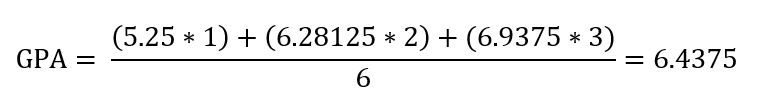For unweighted, the calculation is as follows: